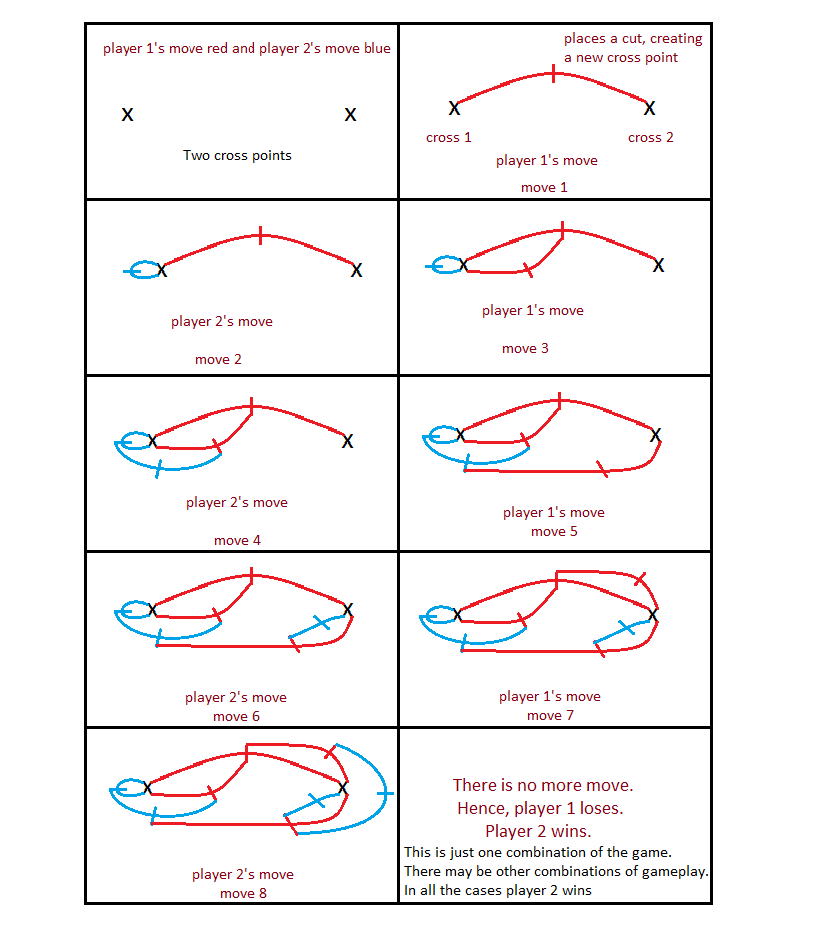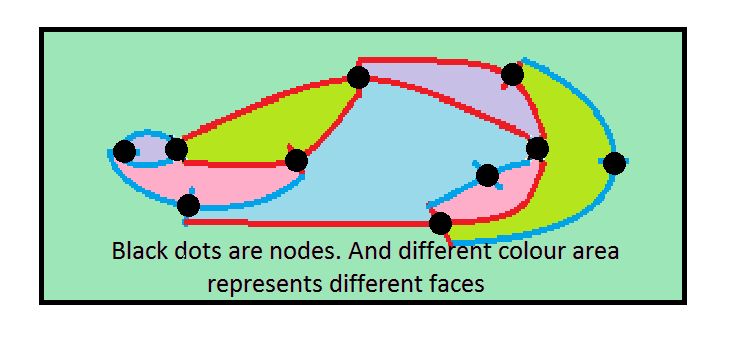# Puzzle | Game of Brussels Sprouts

Given n number of spots, and two players. Players need to connect any two spots without intersecting any of the drawn line, the player who cannot give a move, loses. Determine who will win player1 or player2.

Examples :

Input : n = 2
Output : player 2

Input : n = 3
Output : player 1


Explanation :## Recommended: Please try your approach on {IDE} first, before moving on to the solution.

Method : The winner of this game does not depend on the moves the players choose, rather it only depends on n. This problem can be solved using Euler Characteristics.

According to Euler Characteristics : For every surface S there exists an integersuch that whenever a graph G with V vertices and E edges is embedded in S so that there are F faces(regions divided by the graph), then :.

For a planar graph.

Consider the final picture(i.e. situation after the last move).Solution Approach using Euler Characteristics:
1. In the final picture we can take each cross point as a vertex.
2. Each line joining these cross points can be considered as an edge and
3. Each area section(including the outer one) can be considered as a face of the graph.

Let, the number of moves possible for a particular n is m. To solve the problem, value of m must be found.

Observations :
1. There are initially n crosses(i.e. n vertices). Each move create one new cross. Hence, at last there will be total V = (n+m) crosses(vertices).
2. In each move as player join two free ends by a line and make a new cross in the line(creating a new vertex), next player end up with two new edges connecting the three vertices(old 2, new 1). Hence, after m moves there will be E = 2*m edges in the graph.
3. It can be seen that there is exactly one free end inside each of the faces. Hence, the number of faces is equal to total number of free ends. Again, in each move player connect two free ends(loss two free ends) and create two new free ends(by making one new cross point). Hence, the total number of free ends remains unchanged. So, total number of free ends initially = Number of faces, F = 4*n.

Now, It can be written as : V – E + F = 2
i.e. (n + m) – 2*m + 4*n = 2.
Solving this equation, m = (5*n – 2)

Now if m is odd player 1 will win and if m is even player 2 will win.

## C++

 // C++ code to find out winner  // of Brussels Sprouts game.  #include  using namespace std;     // Function to find out winner of  // the game.  void winner(int n)  {         // Total number of moves m.      int m = 5 * n - 2;         // If m is odd Player 1 is winner      // If m is even Player 2 is winner.      if (m % 2 == 1)          cout << "Player 1" << endl;      else         cout << "Player 2" << endl;  }     // Driver code  int main()  {      // Test case 1      int n1 = 2;      winner(n1);         // Test case 2      int n2 = 3;      winner(n2);         return 0;  }

## Java

 // Java code to find out winner  // of Brussels Sprouts game.     import java.io.*;     class GFG {             // Function to find out winner of      // the game.      static void winner(int n)      {             // Total number of moves m.          int m = 5 * n - 2;             // If m is odd Player 1 is winner          // If m is even Player 2 is winner.          if (m % 2 == 1)              System.out.println("Player 1");          else             System.out.println("Player 2");      }         // Driver code      public static void main(String[] args)      {             // Test case 1          int n1 = 2;          winner(n1);             // Test case 2          int n2 = 3;          winner(n2);      }  }     // This code is contributed by vt_m.

Output:

Player 2
Player 1


My Personal Notes arrow_drop_upI am an undergrad at IIEST Shibpur love to code and solve algorithm data structure problems

If you like GeeksforGeeks and would like to contribute, you can also write an article using contribute.geeksforgeeks.org or mail your article to contribute@geeksforgeeks.org. See your article appearing on the GeeksforGeeks main page and help other Geeks.

Please Improve this article if you find anything incorrect by clicking on the "Improve Article" button below.

Article Tags :
Practice Tags :

Be the First to upvote.

Please write to us at contribute@geeksforgeeks.org to report any issue with the above content.